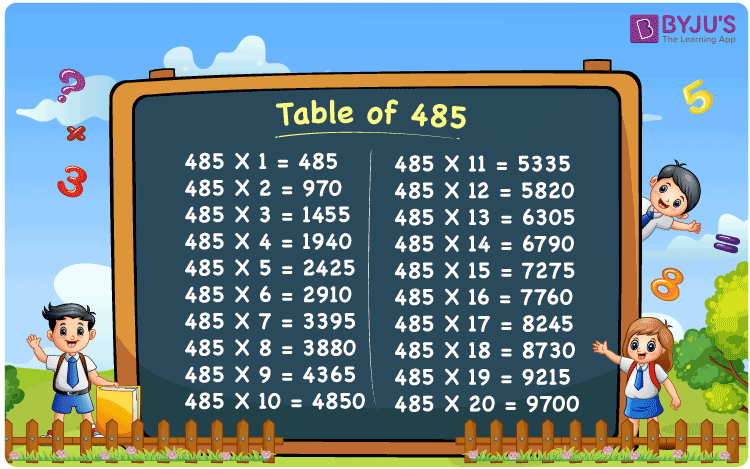Checkout JEE MAINS 2022 Question Paper Analysis : Checkout JEE MAINS 2022 Question Paper Analysis :

# Table of 485

The Table of 485 is given here to help students quickly check the values of the 485 times table up to 20. The multiplication table of 485 is given below in a tabular format, and a PDF is also provided to download. They can download the printable PDF and use the image as a flashcard, which will benefit them to memorise the 485 times table.

## Table of 485 Chart

The table chart of 485 comes in handy for a quick check with the values of multiples of 485. The printable image for the table of 485 can be used as a flashcard and is valuable for doing mental maths calculations, and solving problems for various assessments.## What is the 485 Times Table?

The 485 times table is the multiplication of 485 with the natural numbers. The 485 times table can be obtained both by multiplication and by repeated addition of 485. The below chart shows the multiplication table of 485, in both multiplication and addition form.

 485×1 = 485 485 485×2 = 970 485 + 485 = 970 485×3 = 1455 485 + 485 + 485 = 1455 485×4 = 1940 485 + 485 + 485 + 485 = 1940 485×5 = 2425 485 + 485 + 485 + 485 + 485 = 2425 485×6 = 2910 485 + 485 + 485 + 485 + 485 + 485 = 2910 485×7 = 3395 485 + 485 + 485 + 485 + 485 + 485 + 485 = 3395 485×8 = 3880 485 + 485 + 485 + 485 + 485 + 485 + 485 + 485 = 3880 485×9 = 4365 485 + 485 + 485 + 485 + 485 + 485 + 485 + 485 + 485 = 4365 485×10 = 4850 485 + 485 + 485 + 485 + 485 + 485 + 485 + 485 + 485 + 485 = 4850

## Multiplication Table of 485

Given below are the first 20 multiples of 485 that are the product of the first 20 natural numbers with 485.

 485 × 1 = 485 485 × 2 = 970 485 × 3 = 1455 485 × 4 = 1940 485 × 5 = 2425 485 × 6 = 2910 485 × 7 = 3395 485 × 8 = 3880 485 × 9 = 4365 485 × 10 = 4850 485 × 11 = 5335 485 × 12 = 5820 485 × 13 = 6305 485 × 14 = 6790 485 × 15 = 7275 485 × 16 = 7760 485 × 17 = 8245 485 × 18 = 8730 485 × 19 = 9215 485 × 20 = 9700

## Solved Examples on the Table of 485

Example 1:

A group of 485 students went to a museum. The entry fare for each person is ₹ 10. What is the total cost of fare for all of them?

Solution:

Fare for each person = ₹ 10

Number of students = 485

Total fare = 485 × 10 = ₹ 4850

Therefore, the total cost of fare for all of them is ₹ 4850.

## Frequently Asked Questions on the Table of 485

### What is the 485 times table?

The 485 times table is the multiplication table of 485 with given natural numbers. For example, 485 × 1 = 485, 485 × 2 = 970, 485 × 3 = 1455, 485 × 4 = 1940, 485 × 5 = 2425 and so on.

### Which times table has 485?

485 comes in the times tables of 1, 5, 97 and 485.

### Is 485 a prime number?

No, 485 is a composite number.